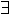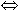Extra sml procedures for ProofPower
Overview
 This document contains extra sml procedures for use with ProofPower to support the methods adopted at X-Logic.org.
 Building Proof Contexts The available proof context facilities are a bit cumbersome if you want to build up a proof context incrementatlly as you work your way through a theory. Here are some hacks to make that easier.
Building Proof Contexts
 The available proof context facilities are a bit cumbersome if you want to build up a proof context incrementatlly as you work your way through a theory. Here are some hacks to make that easier.
force new theory
This procedure is for making a new theory in a context where a previous version of the same theory could be present and if so should be deleted (even if it has children).
 xl-sml fun force_new_theory name = let val _ = force_delete_theory name handle _ => (); in new_theory name end;

force new proof context
This procedure is for making a new pc in a context where a previous version of the pc could be present and if so should be deleted.
 xl-sml fun force_new_pc name = let val _ = delete_pc name handle _ => (); in new_pc name end;

 xl-sml fun add_pc_thms pc thms =(add_rw_thms thms pc;add_sc_thms thms pc;add_st_thms thms pc);
 xl-sml open_theory "basic_hol"; val xl_consistency_result = ref t_thm; fun xl_cs__conv x =if x =\$ (concl(!xl_consistency_result))then_t_intro (!xl_consistency_result)else eq_sym_conv x; force_new_pc "xl_cs__conv"; set_cs__convs [xl_cs__conv] "xl_cs__conv"; set_pr_conv basic_prove_conv "xl_cs__conv"; set_pr_tac basic_prove_tac "xl_cs__conv"; commit_pc "xl_cs__conv"; fun xl_set_cs__thm thm = (xl_consistency_result := thm);